Understanding Fixed-Income Risk and Return | IFT World
IFT Notes for Level I CFA® Program
IFT Notes for Level I CFA® Program

# Part 1

## 1.  Introduction

• Sources of return on a bond: reinvestment of coupon payments and receipt of principal.
• How investors are exposed to different interest rate risk if the same bond is held for different time periods.
• Duration and convexity as measures of interest rate risk.
• How duration and convexity can be used to predict a change in a bond’s price, and assess credit and liquidity risks.

## 2.  Sources of Return

The total return is the future value of reinvested coupon interest payments and the sale price (or redemption of principal if the bond is held to maturity). The horizon yield (or holding period rate of return) is the internal rate of return between the total return and the purchase price of the bond.

Total return on a bond = reinvested coupon interest payments + sale/redemption of principal at maturity

A bond investor has three sources of return:

• Receiving the full coupon and principal payments on the scheduled dates.
• Reinvesting the interest payments. This is also known as interest-on-interest.
• Potential capital gain or loss on sale of the bond, if the bond is sold before maturity date.

Now, we will look at a series of examples that demonstrate the effect on two investors’ realized rate of returns when one of these variable changes: time horizon, interest rate at which the coupons are reinvested, market discount rates at the time of purchase and at the time of sale.

In Examples 1 and 2, we look at the realized rate of return for two investors with different time horizons for the same bond.

Example 1: Calculating the total return on a bond that is held until maturity

Investor 1: A “buy-and-hold” investor purchases a 5 year, 10% annual coupon payment bond at 92.79 per 100 of par value and holds it until maturity.  Calculate the total return on the bond.

Solution: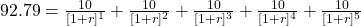Use the following keystrokes to calculate the bond’s yield to maturity:

N = 5; PV = -92.79; PMT = 10; FV = 100; CPT I/Y;

Hence, r = 12%.

This is the yield-to-maturity at the time of purchase. However, this holds good only if all of the following three conditions are true:

• The bond is held to maturity.
• The coupon and final principal payments are made on time (no default or delay).
• The coupon payments are reinvested at the same rate of interest.

To calculate the total return on the bond, we first need to calculate the interest earned when coupon payments are reinvested.

Coupon reinvestment:

• The investor receives 5 coupon payments of 10 (per 100 of par value) for a total of 50, plus the redemption of principal (100) at maturity. The investor has the opportunity to reinvest the cash flows. If the coupon payments are reinvested at 12% (i.e. yield to maturity), the future value of the coupons on the bond’s maturity date is 63.53 per 100 of par value.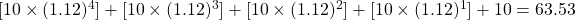• The first coupon payment of 10 is reinvested at 12% for 4 years until maturity; the second is reinvested for 3 years and so on. The future value of the annuity is obtained easily using a financial calculator: N = 5; PV = 0; PMT = 10; I/Y = 12; CPT FV. FV = -63.53
• The amount in excess of the coupons, 13.53 (= 63.53 – 50), is the ‘interest-on-interest’ gain from compounding.
• The investor’s total return is 163.53, the sum of the reinvested coupons (63.53) and the redemption of principal at maturity (100). The realized rate of return is 12%.
92.79 =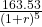, r=12%

Example 2: Calculating the total return on a bond that is sold before maturity

Now let us consider investor 2 who buys the same 5-year, 10% annual coupon payment bond but sells the bond after three years. Assuming that the coupon payments are reinvested at 12% for three years, calculate the total return on the bond.

Solution:

The future value of the reinvested coupons is 33.74 per 100 of par value.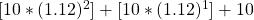= 33.74

The interest-on-interest gain from compounding is 3.74 (= 33.74 – 30). After three years, when the bond is sold, it has two years remaining until maturity. If the yield-to-maturity remains 12%, then the sale price of the bond is 96.62.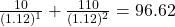The total return is 130.36 (= 33.74 + 96.62) and the realized rate of return is 12%.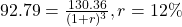This ‘r’ is called the horizon yield, the internal rate of return between the total return and the purchase price of the bond. Horizon yield is equal to the original yield-to-maturity if:

• Coupon payments are reinvested at the same yield-to-maturity calculated at the time of purchase of the bond. In our case, it is 12% as calculated in Example 1.
• There are no capital gains or losses when the bond is sold. It is sold at a price on the constant-yield price trajectory. We arrive at the price 96.62 by taking 12% as the constant yield for the remaining two years. If the yield is more than 12%, then losses occur. Similarly, if the yield is less than 12%, then capital gains occur. This concept is elaborated below:

Constant-yield price trajectory for a 5-year, 10% annual payment bond

The price of the bond at different time periods for a yield of 12% is plotted in the graph below:

The calculations for determining the price are shown below:

 Year Price Calculation 0 92.79 N = 5 ; I/Y = 12 ; PMT = 10 ; FV = 100 ; CPT PV 1 93.93 N = 4 ; I/Y = 12 ; PMT = 10 ; FV = 100 ; CPT PV 2 95.19 N = 3 ; I/Y = 12 ; PMT = 10 ; FV = 100 ; CPT PV 3 96.62 N = 2 ; I/Y = 12 ; PMT = 10 ; FV = 100 ; CPT PV 4 98.21 N = 1 ; I/Y = 12 ; PMT = 10 ; FV = 100 ; CPT PV 5 100

Observation:

There will be a capital gain if the bond is sold at a price above the trajectory at any point in time during the bond’s life. This will happen if the yield is below 12%. Remember a bond’s price and interest rates are inversely related. Similarly, there will be a capital loss if the bond is sold at a price below the trajectory at any point in time during the bond’s life. This will happen if the yield is above 12%. Any point on the trajectory represents the carrying value of the bond at that time.

Next, we analyze what happens to the investors’ realized rate of return if interest rates go up after the bond is purchased.

Example 3: Calculating an investor’s realized return when interest rates go up and bond is held until maturity

The buy-and-hold investor purchases the same 5-year, 10% annual payment bond at 92.79. After the bond is purchased and before the first coupon is received, interest rates go up to 15%.

Calculate the investor’s realized rate of return.

Solution:

Use the following keystrokes to calculate the future value of the reinvested coupons at 15% for 5 years: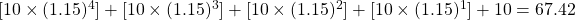The total return is 167.42 (= 67.42 + 100).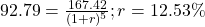The investor’s realized rate of return is 12.53%.

Observation:

Compared to the investor in Example 1 (12%), the realized return of this investor is higher because the coupons are reinvested at a higher rate. There is no capital gain or loss because the bond is held to maturity and the principal of 100 is redeemed.

Example 4: Calculating an investor’s realized return when interest rates go up and bond is sold before maturity

The second investor buys the same 5-year, 10% annual payment bond at 92.79 and sells it in three years. After the bond is purchased, interest rates go up to 15%. Calculate the investor’s realized gain.

Solution:

The future value of the reinvested coupons at 15% after three years is: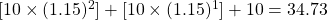N = 3; PV = 0; PMT = 10; I/Y = 15; CPT FV; FV = -34.73

The sale price of the bond after three years is 91.87.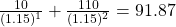The total return is 126.60 (= 34.73 + 91.87), resulting in a realized three-year horizon yield of 10.91%.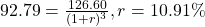N = 3; PV = 92.79; FV = 126.60; CPT I/Y
Hence, r = 10.91%.

Observation:

Compared to the investor in Example 2 with a similar time horizon (12%), the realized return to this investor is lower at 10.91% because there is a capital loss. Even though the coupons are reinvested at a higher rate, the capital loss is greater than the gain from reinvesting coupons.

Increase in the value of reinvested coupons = 34.73 – 33.74  = 0.99

Capital loss = 91.87 – 96.62 = -4.75. Capital gain or loss is always calculated relative to the carrying value at that point in time.

Next, we look at the effect of realized returns when interest rates go down after the bond is issued.

Example 5: Calculating an investor’s realized return when interest rates go down

The buy-and-hold investor purchases the same 5-year, 10% annual payment bond at 92.79 and holds the security until it matures. After the bond is purchased and before the first coupon is received, interest rates go down to 8%. Calculate the investor’s realized return.

Solution:

The future value of reinvesting the coupon payments at 8% for 5 years is 58.67 per 100 of par value.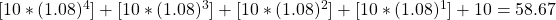The total return is 158.67 (= 58.67 + 100), the sum of the future value of reinvested coupons and the redemption of par value.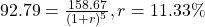The investor’s realized rate of return is 11.33%.

Observation:

The realized return is lower than that in Example 1 (12%) because the coupons are reinvested at a lower rate of return. Since the bond is held to maturity, there is no capital gain or loss.

Decrease in the value of reinvested coupons = 58.67 – 63.53 = – 4.86

Example 6: Calculating an investor’s realized return when interest rates go down

The second investor buys the same 5-year, 10% annual payment bond at 92.79 and sells it after three years. After the bond is purchased, interest rates go down to 8%. Calculate the investor’s realized return.

Solution:

The future value of the reinvested coupons at 8% after three years is: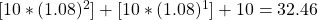This reduction in the future value of coupon reinvestments is offset by the higher sale price of the bond, which is 103.57 per 100 of par value.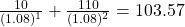The total return is 136.03 (= 32.46 + 103.57), resulting in a realized three-year horizon yield of 13.60%.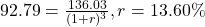Observation:

The realized return is greater than that of the investors in Examples 2 and 4 with a similar time horizon. It is primarily due to the capital gains.

Capital gain = 103.57 – 96.62 = 6.95

Decrease in the value of reinvested coupons = 32.46 – 33.74 = -1.28

As you can see, the capital gain is far greater than the decrease in the value of reinvested coupons.

Interest rate risk affects the realized rate of return for any bond investor in two ways: coupon reinvestment risk and market price risk. But, what is interesting is that these are offsetting types of risk. Two investors with different time horizons will have different exposures to interest rate risk. From the examples above, let us sum up what happens when interest rates go up or down:

When interest rates go up or down:

• Reinvestment income is directly proportional to interest rate movements. The value of reinvested coupons increases when the interest rate goes up.
• Bond price is inversely proportional to interest rate movements. Bond price decreases when the interest rate goes up.

When does coupon reinvestment risk matter?

• When an investor has a long term horizon. If the investor buys a bond and sells it before the first coupon payment, then this risk is irrelevant. But a buy-and-hold investor as in Examples 1, 3 and 5 has only coupon reinvestment risk.

When does market price risk matter?

• Market price risk matters when an investor has a short-term horizon relative to the time-to-maturity. If the investor buys a bond and sells it before the first coupon payment, then there is only market price risk. But a buy-and-hold investor such as those in Examples 1, 3 and 5 has only coupon reinvestment risk, and no market price risk.

Example 7: Calculating the purchase price of the bond for various YTM

An investor buys a five-year, 10% annual coupon payment bond priced to yield 8%. The investor plans to sell the bond in three years once the third coupon payment is received. Calculate the purchase price for the bond and the horizon yield assuming that the coupon reinvestment rate after the bond purchase and the yield-to-maturity at the time of sale are (1) 7% (2) 8% and (3) 9%.

Solution:

The purchase price is: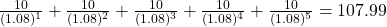N = 5; PMT = 10; FV = 100; I/Y = 8; CPT PV, PV = -107.99.

Solution to 1:

With YTM at sale = 7%:

N = 3; PV = 0; PMT = 10; I/Y = 7; CPT FV, FV = -32.15.

The future value of the reinvested coupons after three years is 32.15.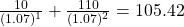N = 2; I/Y = 7; PMT = 10; FV = 100; CPT PV, PV = 105.42.

The sale price of the bond after three years is 105.42.

The total return after three years is 137.57 (= 32.15 + 105.42).

The realized return calculated using the keystrokes: N = 3; PMT = 0; PV = 107.99; FV = 137.57; CPT I/Y,

r = 8. 4%.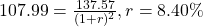If interest rates go down from 8% to 7%, then the realized rate of return over the three-year investment horizon is 8.40%, higher than the original yield to maturity of 8%.

Solution to 2:

With YTM at sale = 8%, the future value of the reinvested coupons after three years is:

N = 3; PV = 0; PMT = 10; I/Y = 8; CPT FV, FV = -32.46.

The sale price of the bond is calculated as:

N = 2, I/Y = 8, PMT = 10, FV = 100; CPT PV

The sale price of the bond after three years is 103.57.

The total return after three years is 136.03 (= 32.46 + 103.57).

The realized return calculated using the keystrokes: N = 3; PMT = 0; PV = -107.99; FV = 136.03; CPT I/Y.

r = 8%.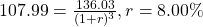If interest rates remain 8% for reinvested coupons and for the required yield on the bond, the realized rate of return over the three-year investment horizon is equal to the yield-to-maturity of 8%.

Solution to 3:

With YTM at sale = 9%: the future value of the reinvested coupons after three years is:

N = 3; PV = 0; PMT = 10; I/Y = 9; CPT FV, FV = -32.78.

The sale price of the bond is calculated as:

N = 2, I/Y = 9, PMT = 10, FV = 100; CPT PV

The sale price of the bond after three years is 101.76.

The total return after three years is 134.54 (= 32.78 + 101.76).

The realized return calculated using the keystrokes: N = 3; PV = -107.99; FV = 134.54; CPT I/Y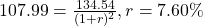;

If interest rates go up from 8% to 9%, the realized rate of return over the three-year investment horizon is 7.60%, lower than the yield to maturity of 8%.

Crash Courses for November CFA Level I, II exams are coming soon!
This is default text for notification bar